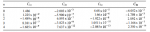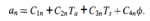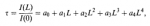# Help solving a fourth order polynominal

#### MathN00b

##### New member
Hello all,

I am trying to use equation 7 & 8 from a fire safety engineering journal to determine the transmittance of a radiant heat flux through the atmosphere - https://ws680.nist.gov/publication/get_pdf.cfm?pub_id=909662

The math seems quite simple and is a fourth order polynomial equation. I have created a spreadsheet to solve the equations.

However, when I have entered the example given in the journal of using an air temperature of T 288K and a relative humidity of 51%, flame temperature of 1300K and path of 200m into my spreadsheet I cannot end up with a transmittance of0.75 as given in the example.

Could someone please advise what they end up with for a0, a1, a2, a3, a4 so ifI can confirm my results. Otherwise, I am happy to provide a link to my spreadsheet if you would like to review.

Any help is much appreciated.

Regards.

#### stapel

##### Super Moderator
Staff member
I am trying to use equation 7 & 8 from a fire safety engineering journal to determine the transmittance of a radiant heat flux through the atmosphere - https://ws680.nist.gov/publication/get_pdf.cfm?pub_id=909662

The math seems quite simple and is a fourth order polynomial equation. I have created a spreadsheet to solve the equations.

However, when I have entered the example given in the journal of using an air temperature of T 288K and a relative humidity of 51%, flame temperature of 1300K and path of 200m into my spreadsheet I cannot end up with a transmittance of0.75 as given in the example.

Could someone please advise what they end up with for a0, a1, a2, a3, a4 so ifI can confirm my results. Otherwise, I am happy to provide a link to my spreadsheet if you would like to review.
Please reply by posting the equation here, and showing your work that led to your answer. Thank you!#### MathN00b

##### New member
Please reply by posting the equation here, and showing your work that led to your answer. Thank you!Sorry for the delayed response.

The constants for the equation are in the following tableThe equation for a1, a2, a3, a4 is as followsContestants from the table above
Ta = 15
Ts = 1023
o = 51

Then the fourth order polynomial iswhere L = 200

I have calculated everything in an excel spreadsheet and will provide a link to it to show my working tomorrow.

#### j-astron

##### Junior Member
Hi MathN00b

You're not "solving" the fourth-order polynomial. That implies finding out where it is equal to 0, which is a very hard problem. You're simply evaluating it at a specific L.

Here is some Python code I wrote up to compute the transmittance from the information in the paper:

Code:
import numpy as np # numerical Python routines

T_a = 288.0 # air temp in kelvins
T_s = 1300.0 # flame temp in kelvins

L = 200.0 # distance source to detector in m
phi = 0.51 # relative humidity

Ncoeffs = 5 # number of a_n values

# Convert the coefficients in Table 1 of Fire Safety Journal 37 (2002)
# 181-190 into a 5 x 4 array:

C = np.array([ [1.486, -2.003e-3, 4.68e-5, -6.052e-2],
[1.225e-2, -5.900e-5, 1.66e-6, -1.759e-3],
[-1.489e-4,  6.893e-7, -1.922e-8, 2.092e-5],
[8.381e-7, -3.823e-9, 1.0511e-10, -1.166e-7],
[-1.685e-9, 7.637e-12, -2.085e-13, 2.350e-10]
])

# Empty array of coefficient values:
a = np.zeros(Ncoeffs)

# Compute the polynomial coefficients (Eq. 8 of paper):
# Note that Python uses zero-indexed, row-major ordering
for n in range(Ncoeffs):

a[n] = C[n] + C[n]*T_a + C[n]*T_s + C[n]*phi

print('Coefficient a_{} = {:.3g}'.format(n, a[n]))

# Compute the transmittance (Eq. 7 of the paper):
tau = a + a*L + a*L**2 + a*L**3 + a*L**4

print('The calculated transmittance is tau = {:.4g}'.format(tau))
and here is the output of that code:

Code:
Coefficient a_0 = 0.939
Coefficient a_1 = -0.00348
Coefficient a_2 = 3.53e-05
Coefficient a_3 = -1.86e-07
Coefficient a_4 = 3.63e-10
The calculated transmittance is tau = 0.7502

#### MathN00b

##### New member
Thank you, this has helped me work out where I was making a mistake. Much appreciated.